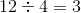# ISEE Middle Level Math : How to find the square root

## Example Questions

### Example Question #41 : Squares / Square Roots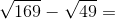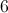Explanation:

First, find the sqaure roots: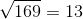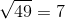Then, solve: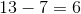### Example Question #42 : Squares / Square Roots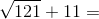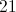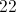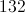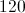Explanation:

First, find the square root: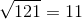Then, solve: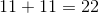### Example Question #43 : Squares / Square Roots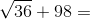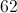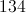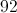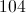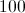Explanation:

First, find the square root: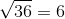Then, solve: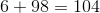### Example Question #44 : Squares / Square Roots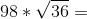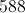Explanation:

First, find the square root:Then, solve: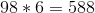### Example Question #45 : Squares / Square Roots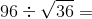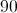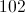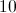Explanation:

First, find the square root:Then, solve: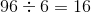### Example Question #46 : Squares / Square Roots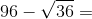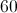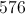Explanation:

First, find the square root:Then, solve: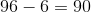### Example Question #47 : Squares / Square Roots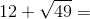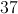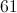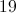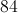Explanation:

First, find the square root:Then, solve: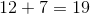### Example Question #48 : Squares / Square Roots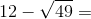Explanation:

First, find the square root:Then, solve: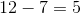### Example Question #49 : Squares / Square Roots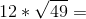Explanation:

First, find the square root:Then, solve: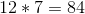### Example Question #50 : Squares / Square Roots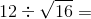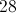Explanation:

First, find the square root: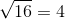Then, solve: